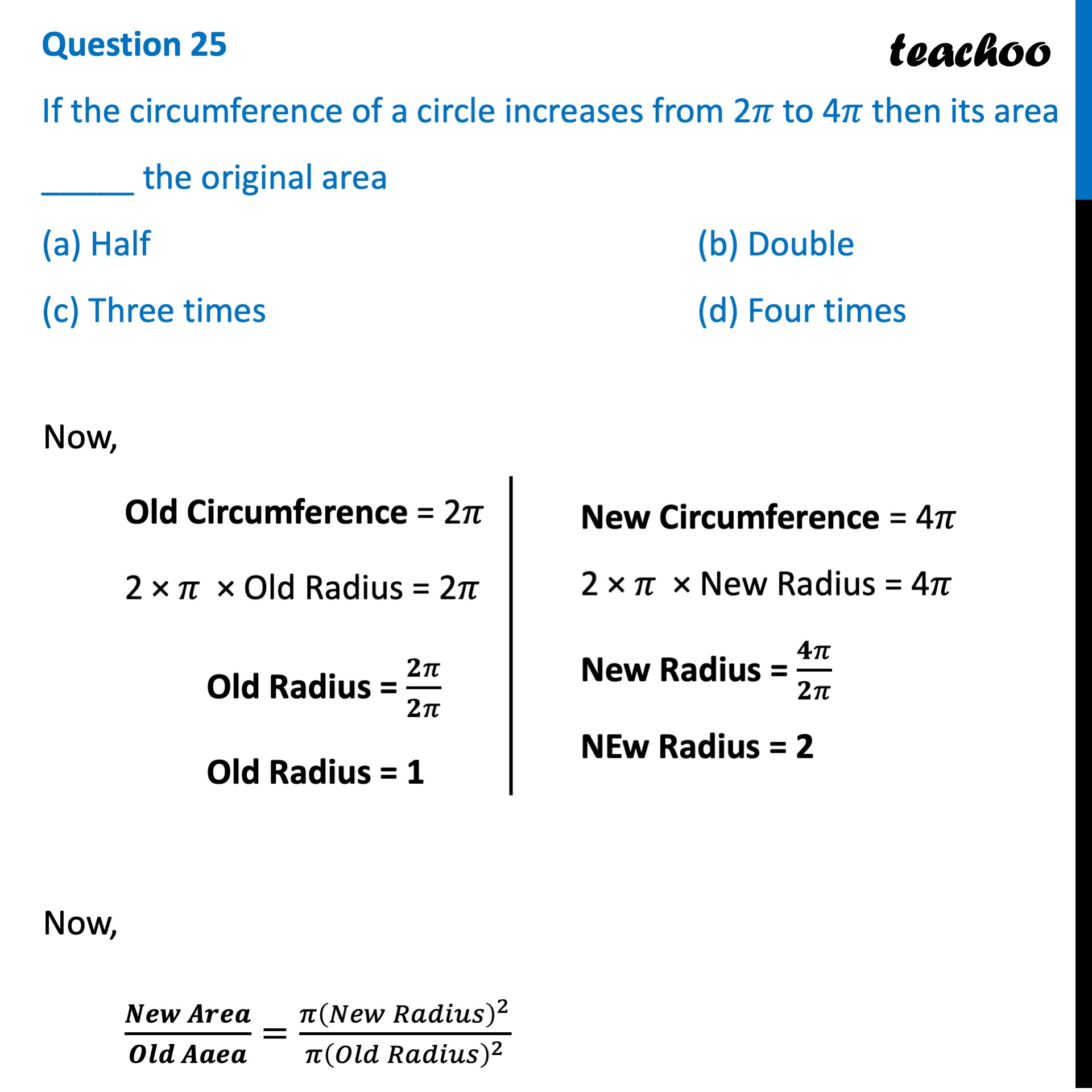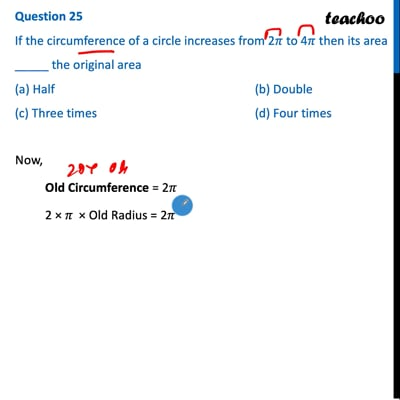CBSE Class 10 Sample Paper for 2022 Boards - Maths Basic [MCQ]

Class 10
Solutions of Sample Papers for Class 10 Boards

## (a) Half   (b) Double  (c) Three times   (d) Four timesThis video is only available for Teachoo black users

Solve all your doubts with Teachoo Black (new monthly pack available now!)

### Transcript

Question 25 If the circumference of a circle increases from 2𝜋 to 4 𝜋 then its area _____ the original area (a) Half (b) Double (c) Three times (d) Four times Now, Old Circumference = 2𝜋 2 × 𝜋 × Old Radius = 2𝜋 Old Radius = 𝟐𝜋/𝟐𝜋 Old Radius = 1 Now, (𝑵𝒆𝒘 𝑨𝒓𝒆𝒂)/(𝑶𝒍𝒅 𝑨𝒂𝒆𝒂)=(𝜋(𝑁𝑒𝑤 𝑅𝑎𝑑𝑖𝑢𝑠)^2)/(𝜋(𝑂𝑙𝑑 𝑅𝑎𝑑𝑖𝑢𝑠)^2 ) New Circumference = 4𝜋 2 × 𝜋 × New Radius = 4𝜋 New Radius = 𝟒𝜋/𝟐𝜋 NEw Radius = 2 =(𝝅 × 𝟐^𝟐)/(𝝅 × 𝟏^𝟐 ) =(𝜋 × 4)/(𝜋 × 1) = 4 Thus, New Area = 4 × Old Area So, the correct answer is (d)